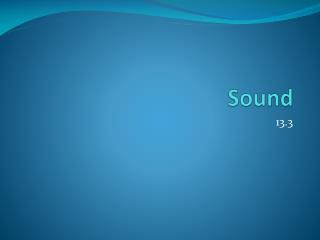DownloadDownload PresentationSound

# Sound

Download Presentation## Sound

- - - - - - - - - - - - - - - - - - - - - - - - - - - E N D - - - - - - - - - - - - - - - - - - - - - - - - - - -
##### Presentation Transcript

1. Sound 13.3

2. Harmonics • A vibrating string will produce standing waves whose frequencies depend upon the length of the string. Harmonics Video 2:34

3. In the lowest frequency of vibration, one wavelength will equal twice the length of string and its called the fundamental frequency (f1). For f1, 1λ = 2L One wavelength = 2*length of string Fundamental Frequency Half of a wavelength

4. Harmonics • A Harmonic series is a series of frequencies that include the fundamental frequency and multiples of that frequency. • 1st harmonic = f1 • 2nd harmonic = f2 = 2*f1 • 3rd harmonic = f3 = 3*f1 • Etc…

5. Harmonics • The second harmonic is the next possible standing wave for the same string length. • This shows an increase in frequency, and a decrease in wavelength. • f2=2f1 λ2 = L Second Harmonic = 2*fundamental frequency

6. Harmonics • As the harmonic increases the frequency increases and wavelength decreases. Ex: • f3= 3f1λ3 = 2/3λ1 • f4= 4f1λ4= ½ λ1 • Standing Waves, Fixed at Both Ends Animation

7. Formula for other harmonics • Harmonic Series of standing waves fn = n* V n=1, 2, 3… 2L Frequency = harmonic number x (speed of waves on the string) (2)*(length of vibrating string)

8. Standing Waves in an Air Column • If both ends of a pipe are open, all harmonics are present and the ends act as antinodes. • This is the exact opposite of a vibrating string, but the waves act the same so we can still use the same formula to calculate frequencies. fn = n* V n=1, 2, 3… 2L Frequency = harmonic number x (speed of waves on the string) (2)*length of vibrating air column)

9. Standing Waves in an Air Column • If one end of the pipe is closed, only odd harmonics are present (1, 3, 5, etc). • This changes the formula: fn = n* V n=1, 3, 5… 4L Frequency = harmonic number*(speed of waves on the string) (4)*length of vibrating air column)

10. Example • What are the first three harmonics in a 2.45 m long pipe that is open at both ends? Given that the speed of sound in air is 345 m/s. • L= 2.45 m v= 345 m/s • fn = n*v/2L • 1st harmonic: f1= 1*(345 m/s)/(2*2.45 m) = 70.4 Hz • 2nd harmonic: f2= 2*(345 m/s)/(2*2.45 m) = 141 Hz • 3rd harmonic: f3= 3*(345 m/s)/(2*2.45 m) = 211 Hz

11. Example • What are the first three harmonics of this pipe when one end of the pipe is closed? Given that the speed of sound in air is 345 m/s. • L= 2.45 m v= 345 m/s • fn = n*v/4L • 1st harmonic: f1= 1*(345 m/s)/(4*2.45 m) = 35.2 Hz • 3rd harmonic: f3= 3*(345 m/s)/(4*2.45 m) = 106 Hz • 5th harmonic: f5= 5*(345 m/s)/(4*2.45 m) = 176 Hz

12. Why do different instruments sound different? • Timbre is the quality of a steady musical sound that is the result of a mixture of harmonics present at different intensities. • This is why a clarinet and a trumpet can play the same pitch but they sound different. • Harmonics Applet

13. Beat • When two waves of slightly different frequencies travel in the same direction they interfere. This causes a listener to hear an alternation between loudness and softness and is called beat.

14. Beat • Formation of Beats Applet • The frequency difference between two sounds can be found by the number of beats per second.